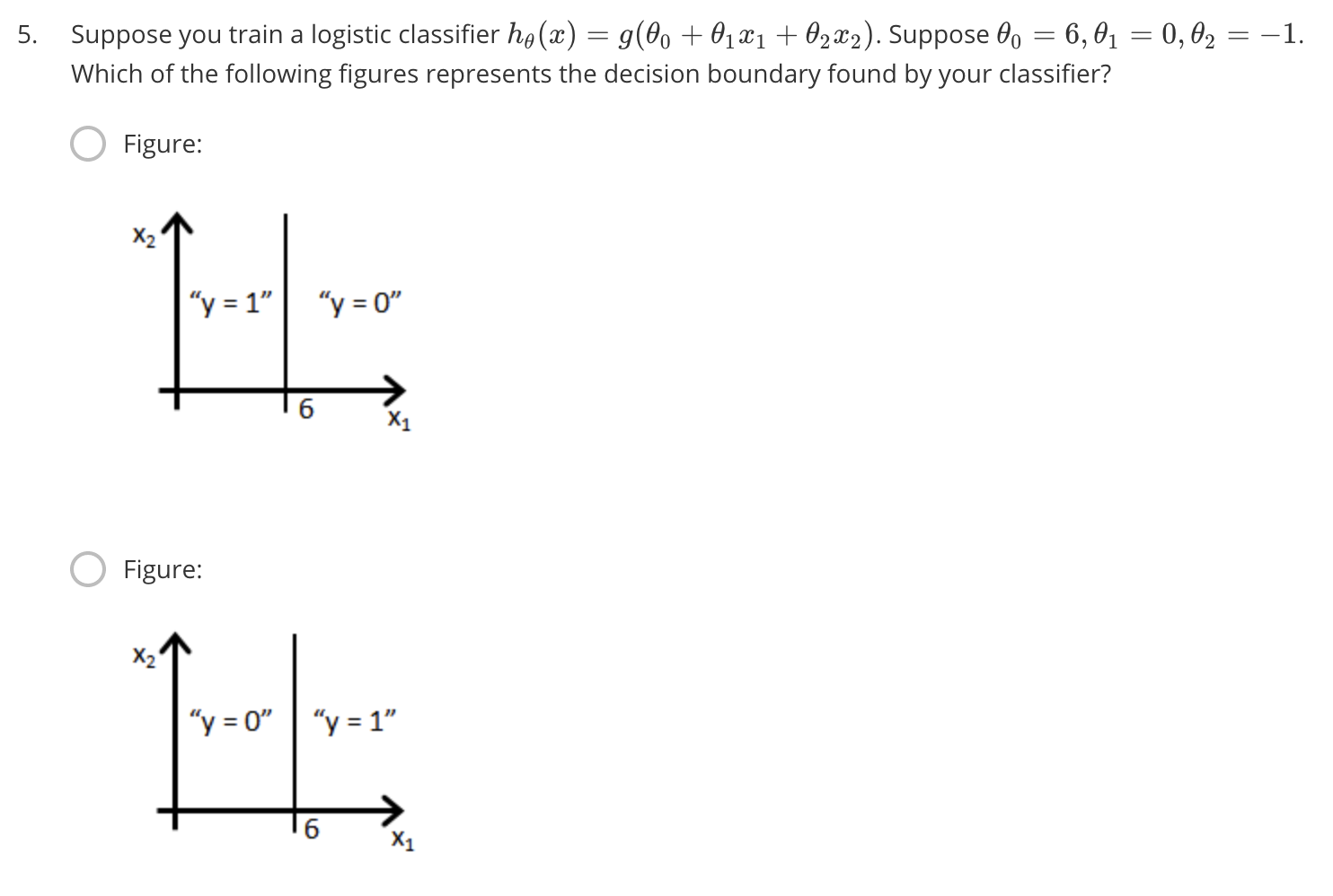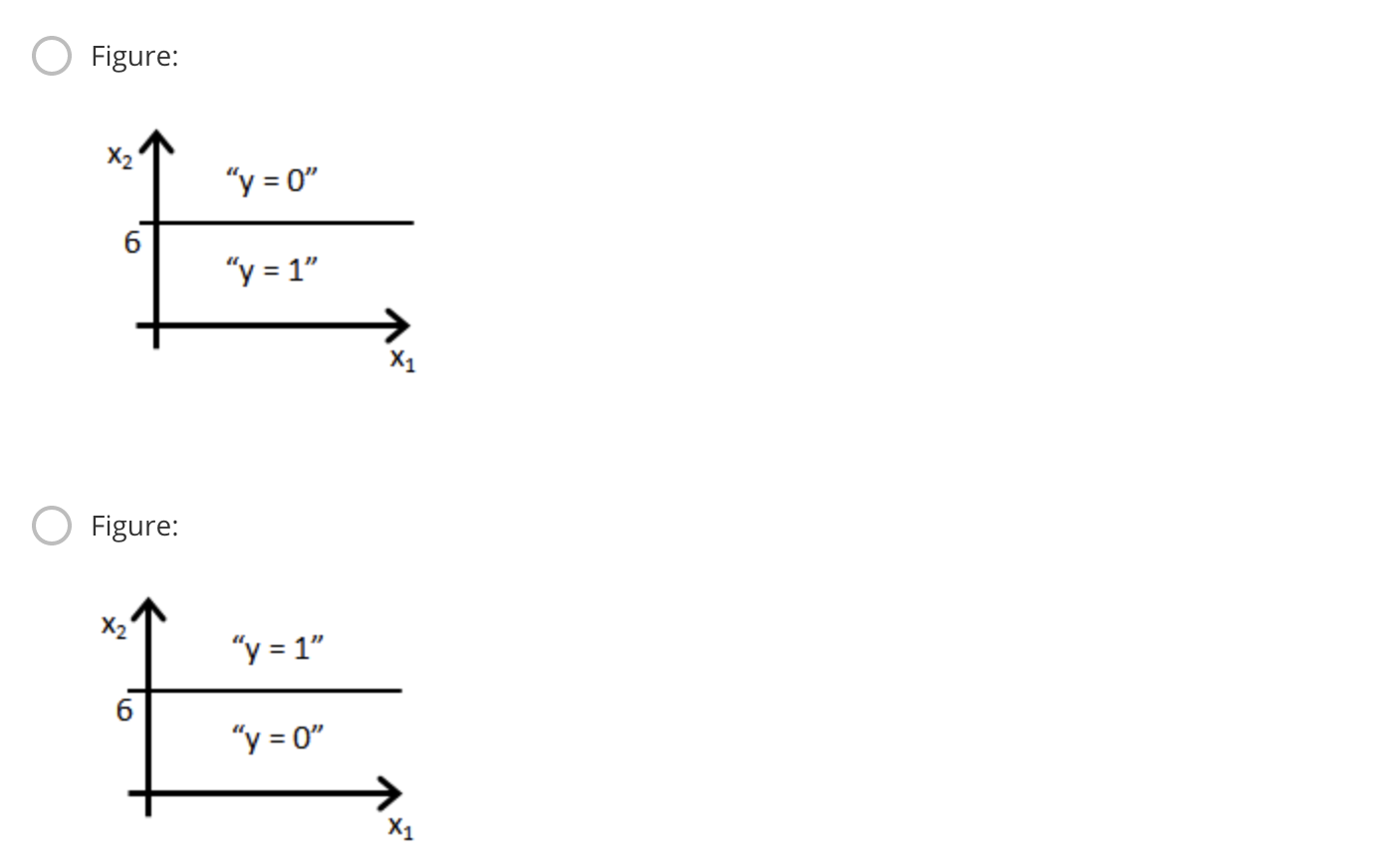## 6.1 Classification and Repressentation

### 6.1.1 Classification

quiz

Which of the following statements is true?

• If linear regression doesn’t work on a classification task as in the previous example shown in the video, applying feature scaling may help.
• If the training set satisfies $0 \le y^{(i)} \le 1$ for every training example
$(x^{(i)}, y^{(i)})$, then linear regression’s prediction will also satisfy $0 \le h_\theta(x) \le 1$ for all values of x.
• If there is a feature $x$ that perfectly predicts $y$, i.e. if $y = 1$ when $x \ge c$ and $y=0$ whenever $x<c$ (for some constant $c$), then linear regression will obtain zero classification error.
• None of the above statements are true.
答案是第四项，以上都不对。

### 6.1.2 Hypothesis Representation

$g(z)$的函数图像为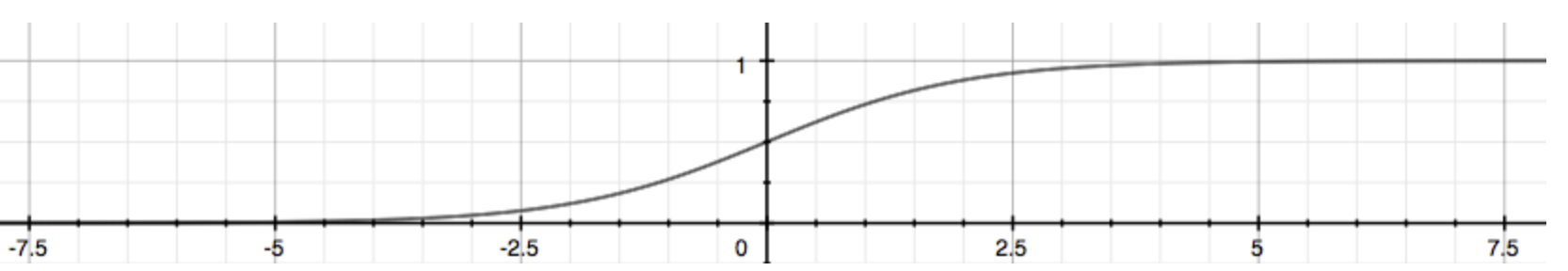### 6.2.1 Decision Boundary

recap 记录

$h\theta(x)$ 的值虽然被映射到 0-1 之间，但是它依然不是 0 或者 1，而是0-1之间的连续数，我们需要指定值，当其大于0.5时，认为分类为1，当其小于0.5时，认为分类值为0。**对于$h\theta(x) = g(\theta^Tx)$其大于或小于0.5，就是 $\theta^Tx$ 大于或小于0。**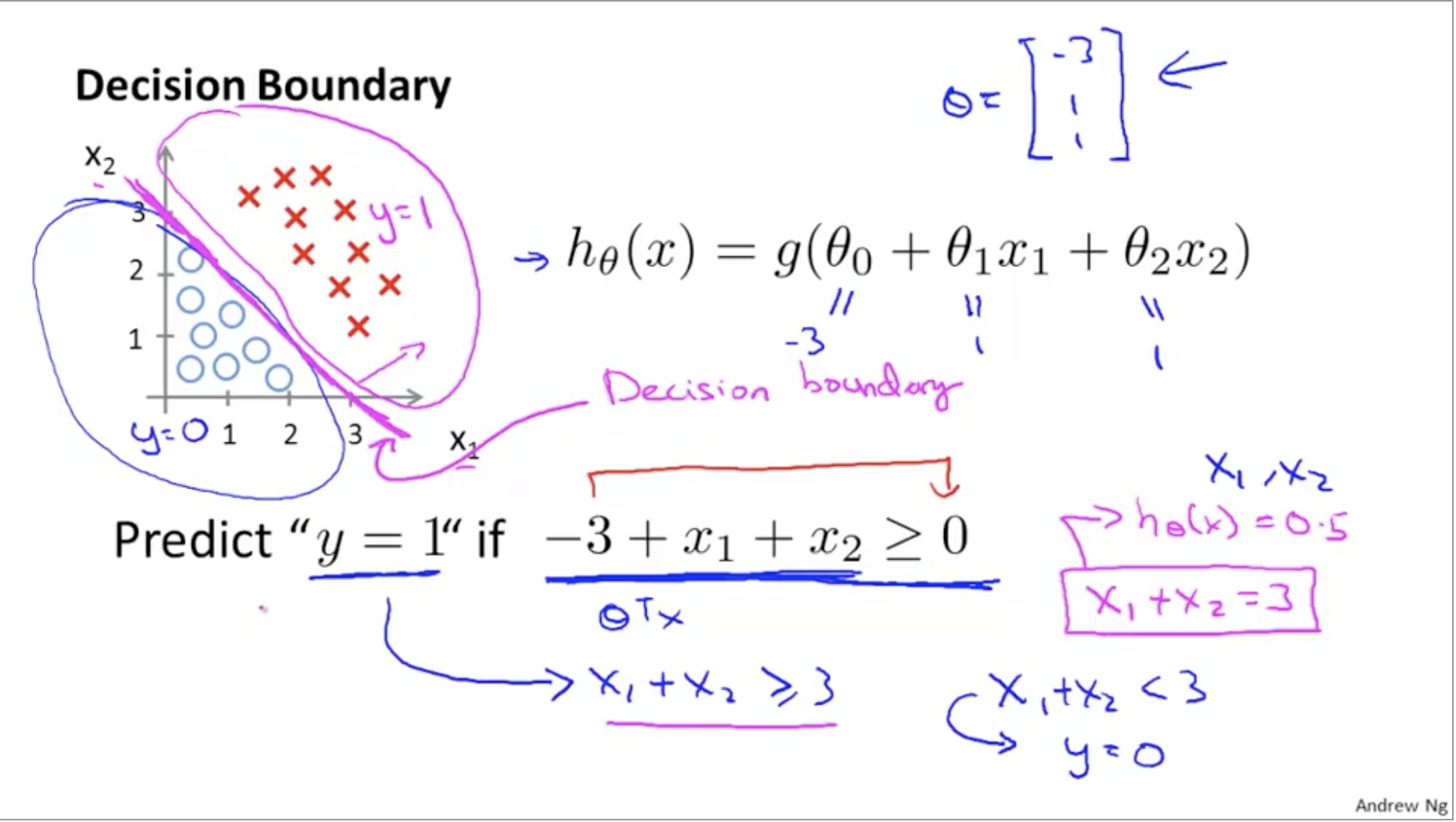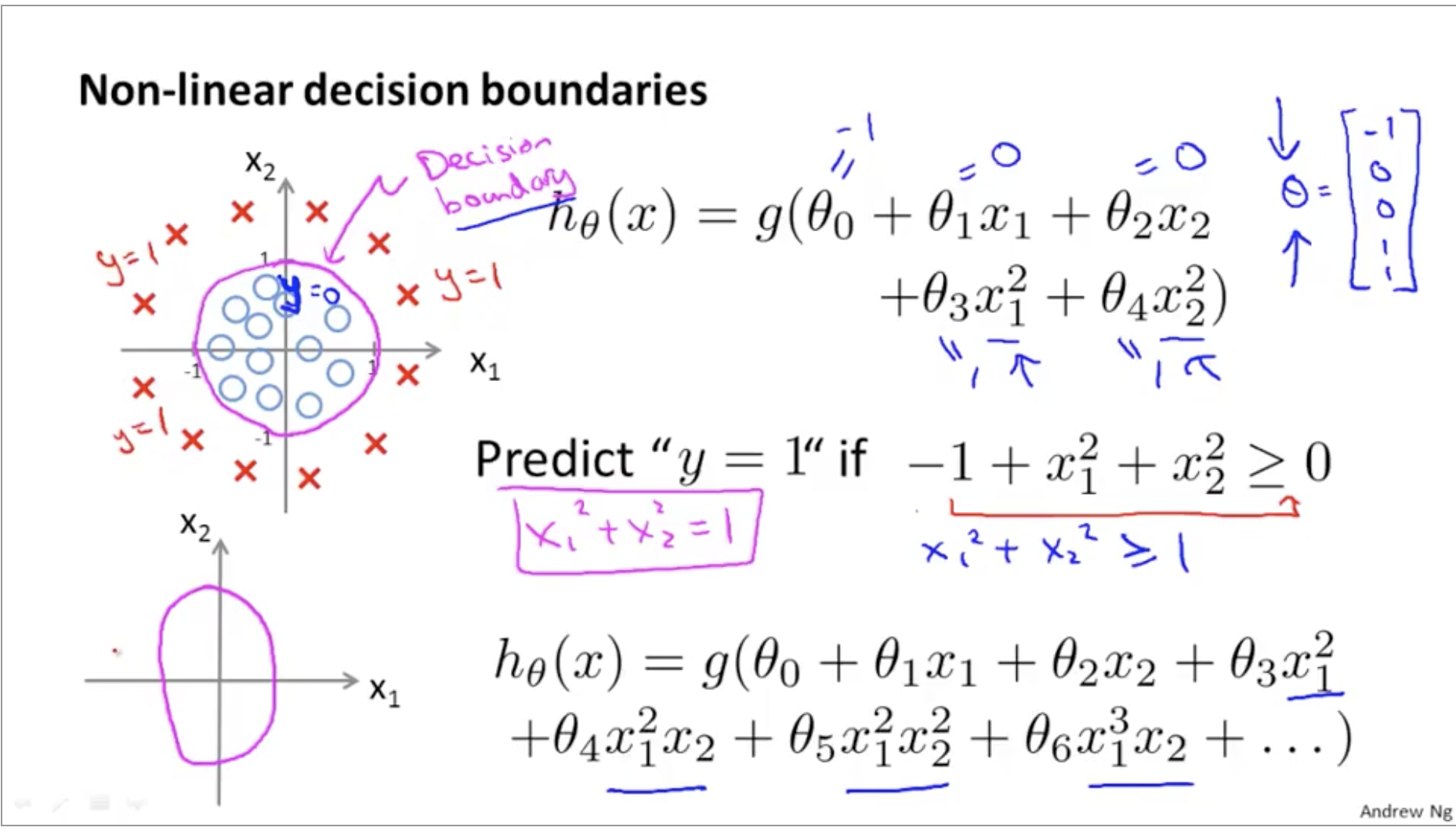## 6.2 Logistic Regression Model

### 6.2.1 Cost Function

convex function 凸函数
non-convex function 非凸函数
nonlinear 非线性
approach to 趋近于

m个样本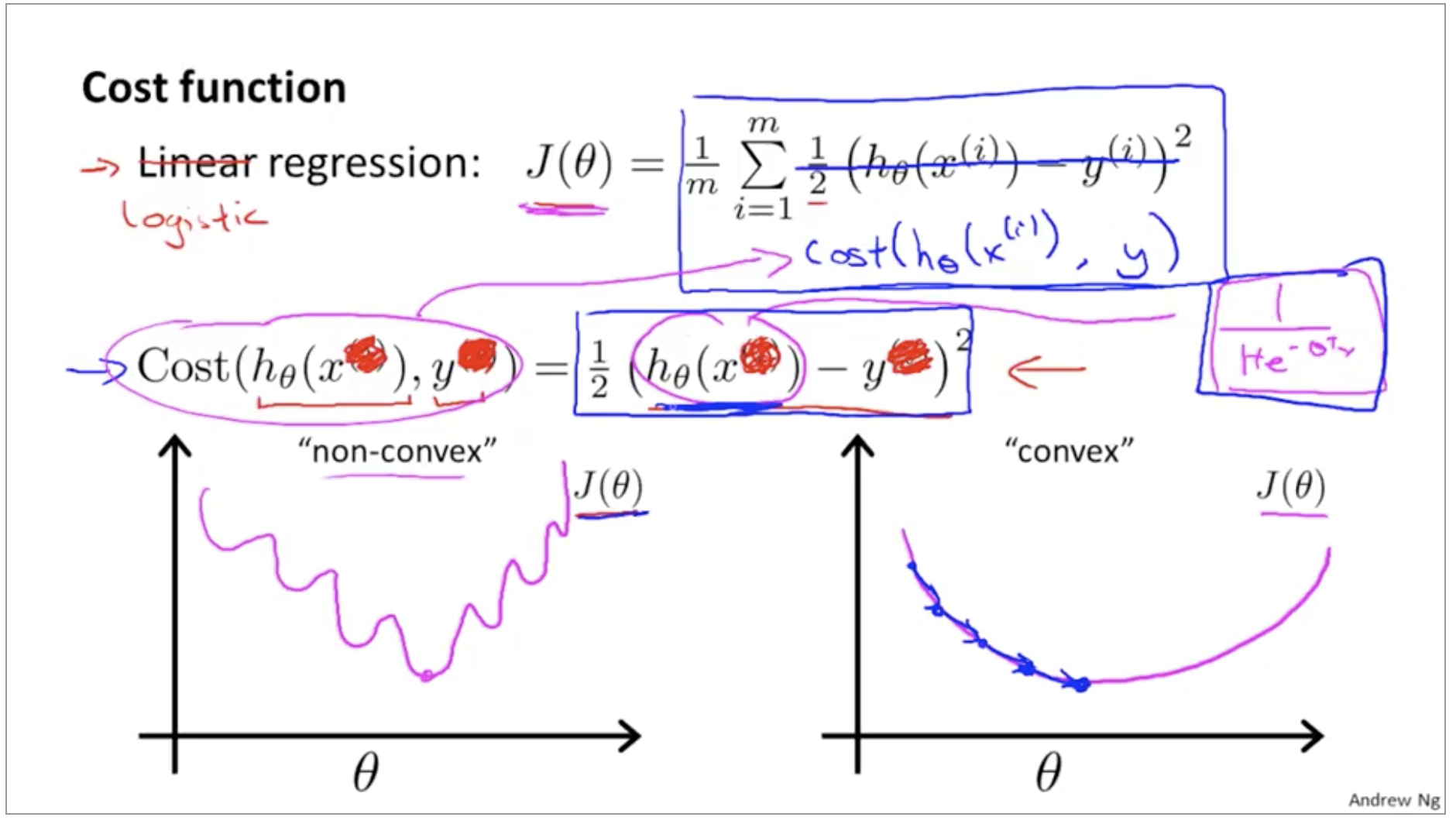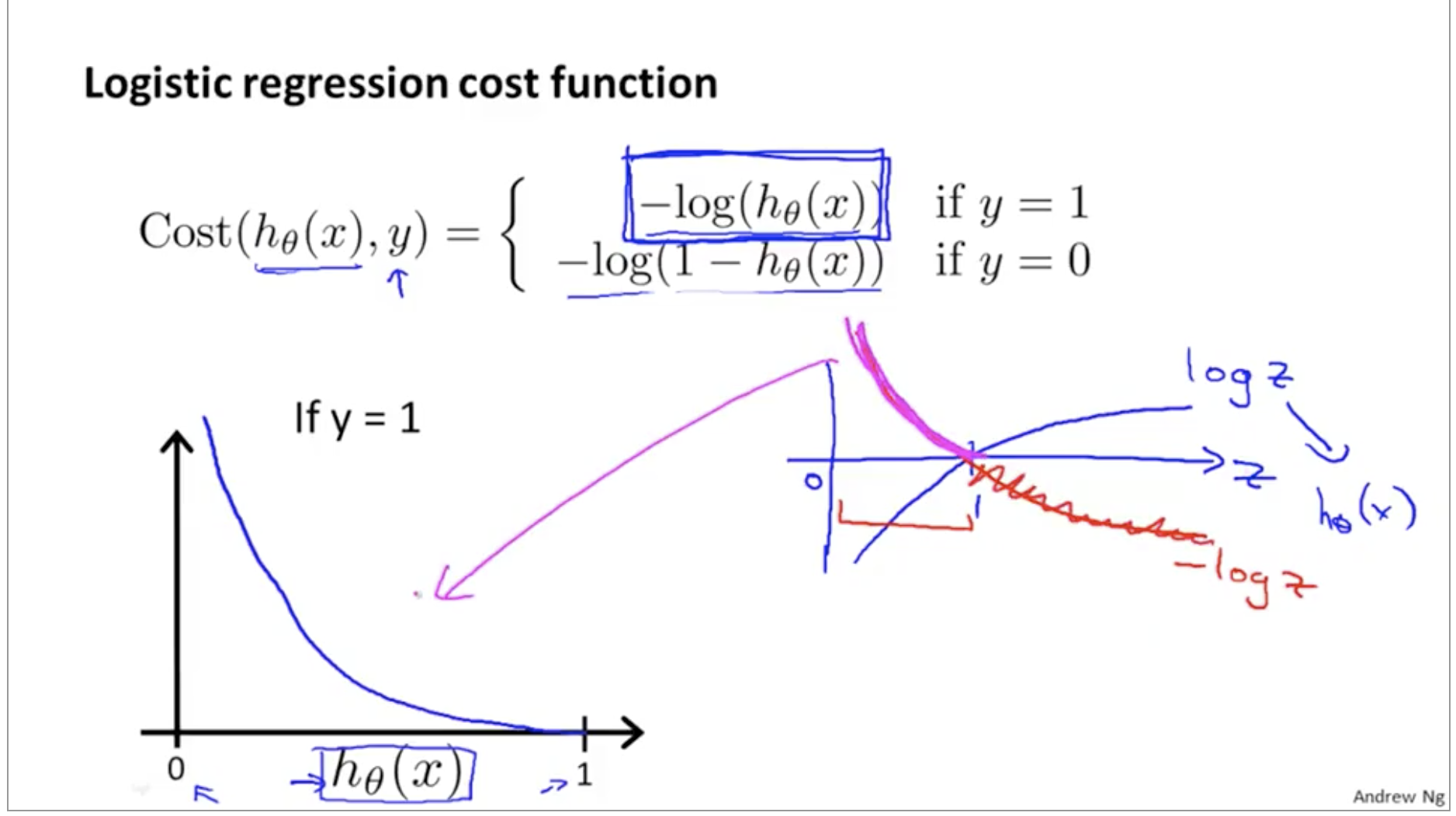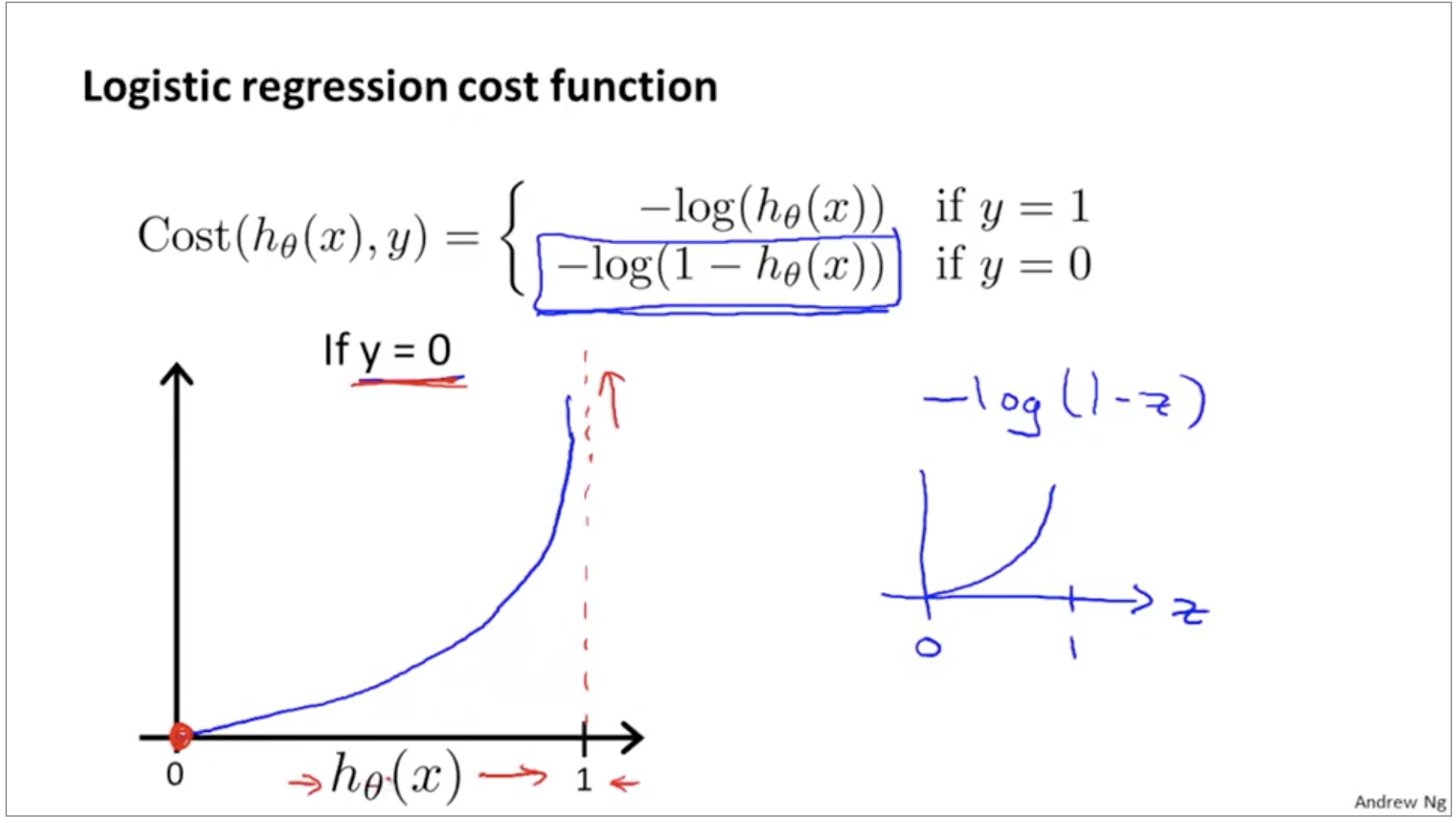quiz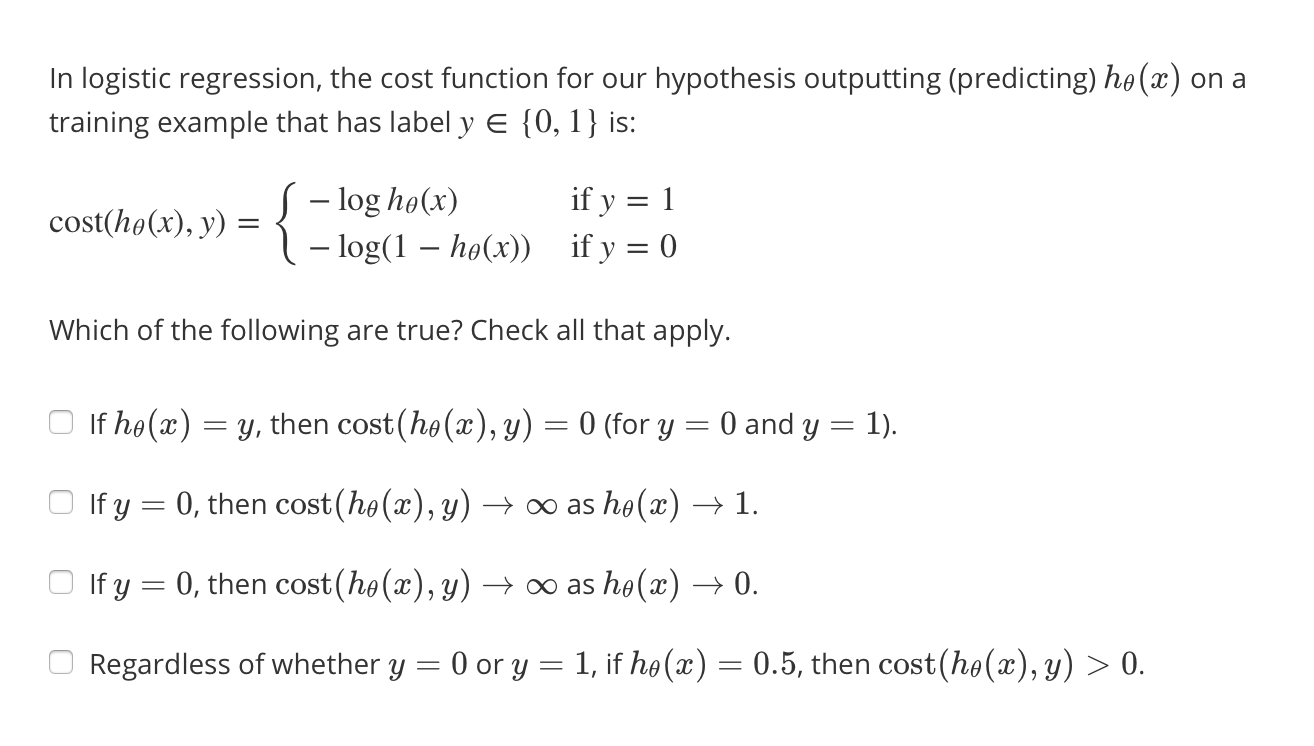### 6.2.2 Simplified Cost Function and Gradient Descent

Note: y = 0 or 1 always

identical 相同的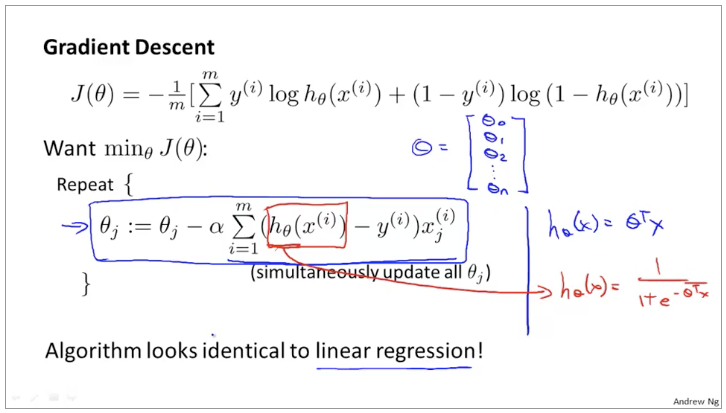• BFGs 变尺度法
• L-BFGs 限制变尺度法

opaque 不透明的

## 6.3 Muticlass Classification

one-vs-rest，对于一个分三类的问题，将其分解成3个二分类问题。分别得出三个判别边界。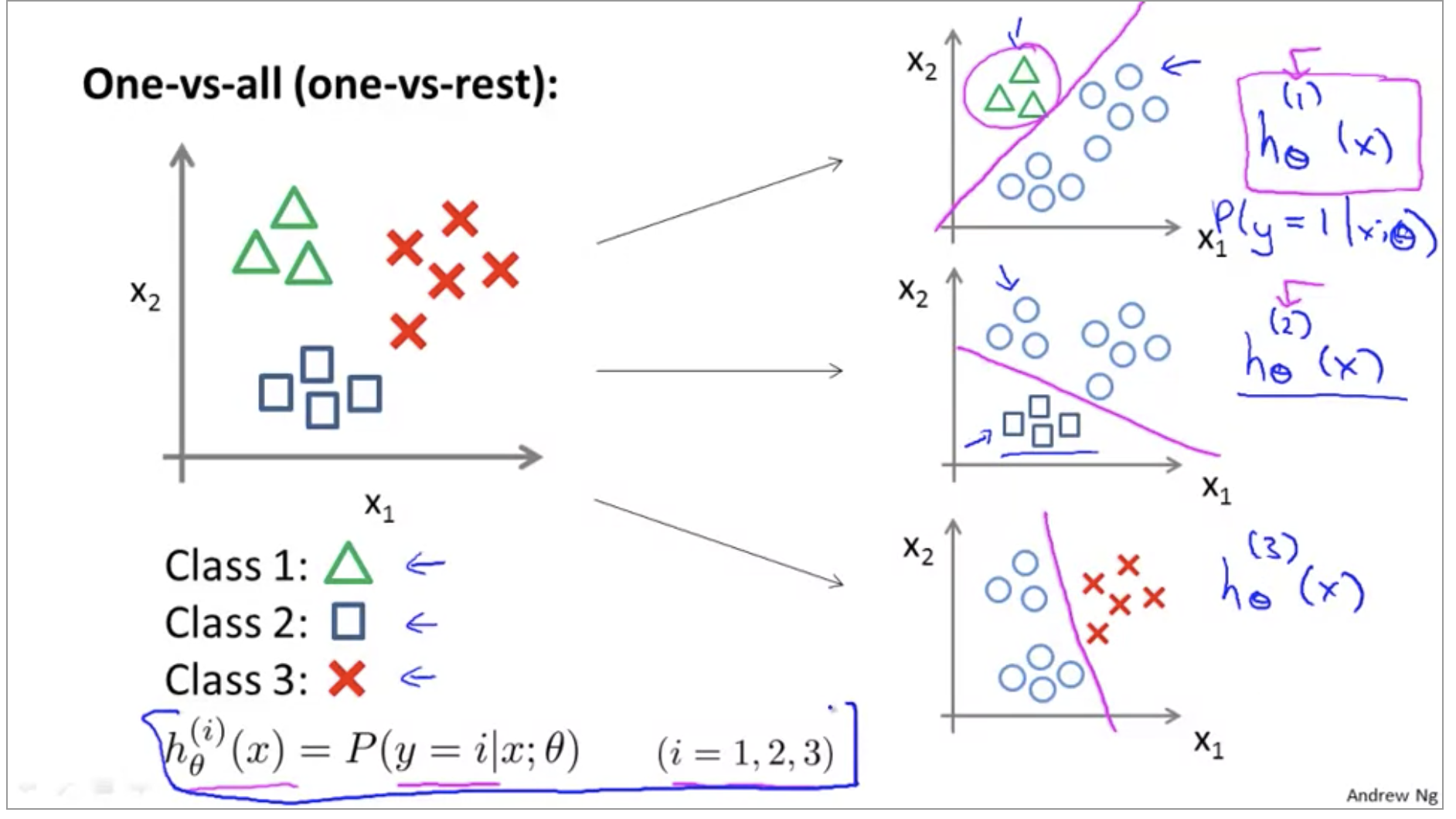## Test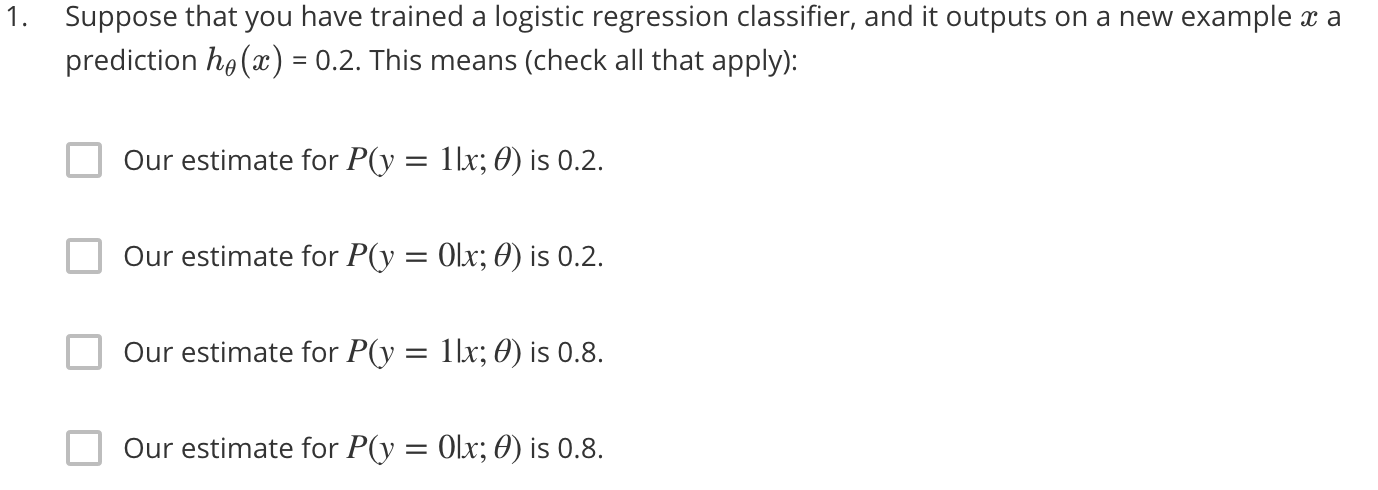1. Suppose you have the following training set, and fit a logistic regression classifier $h_\theta(x) +g(\theta_0+\theta_1x_1+\theta_2x2)$.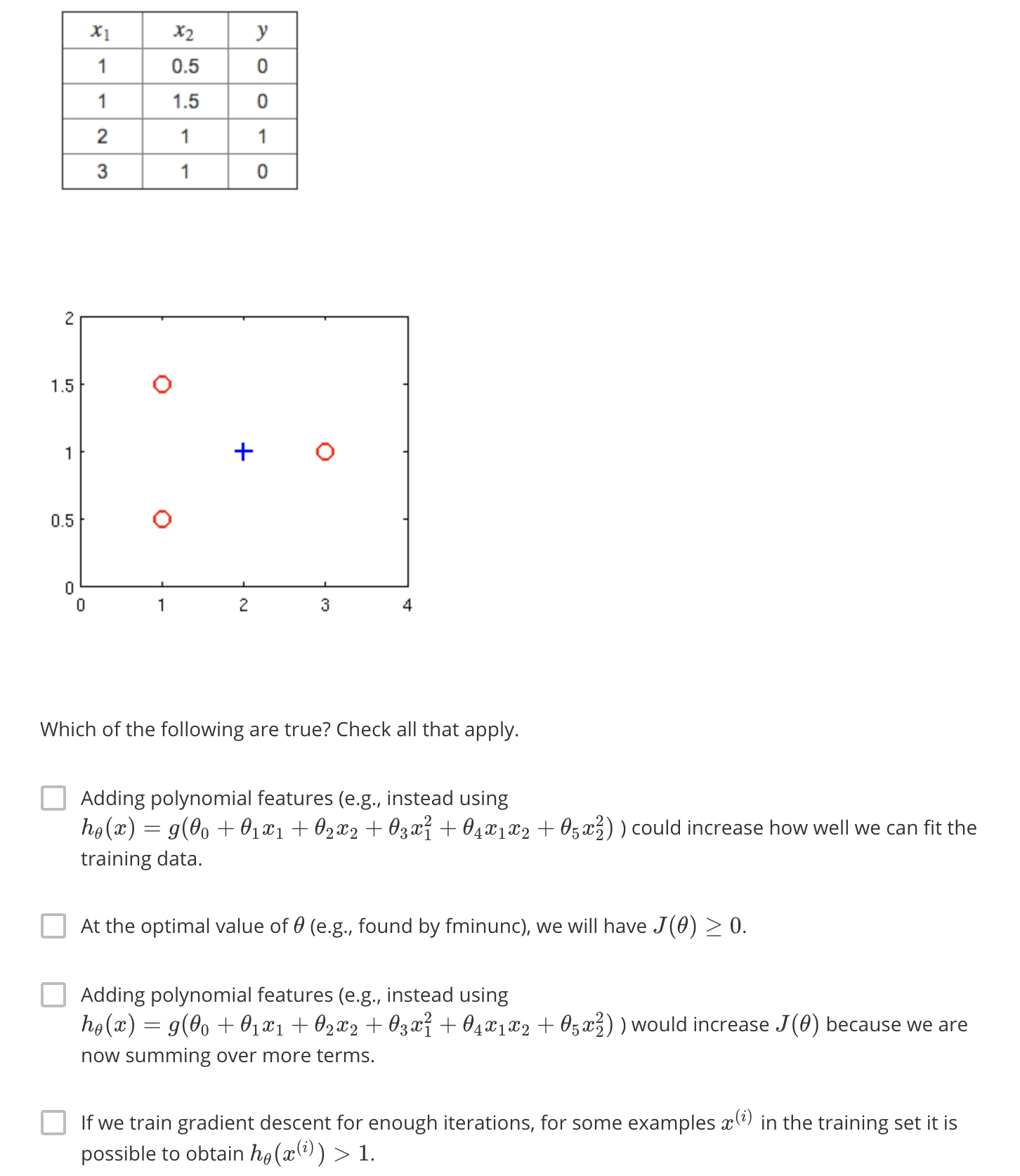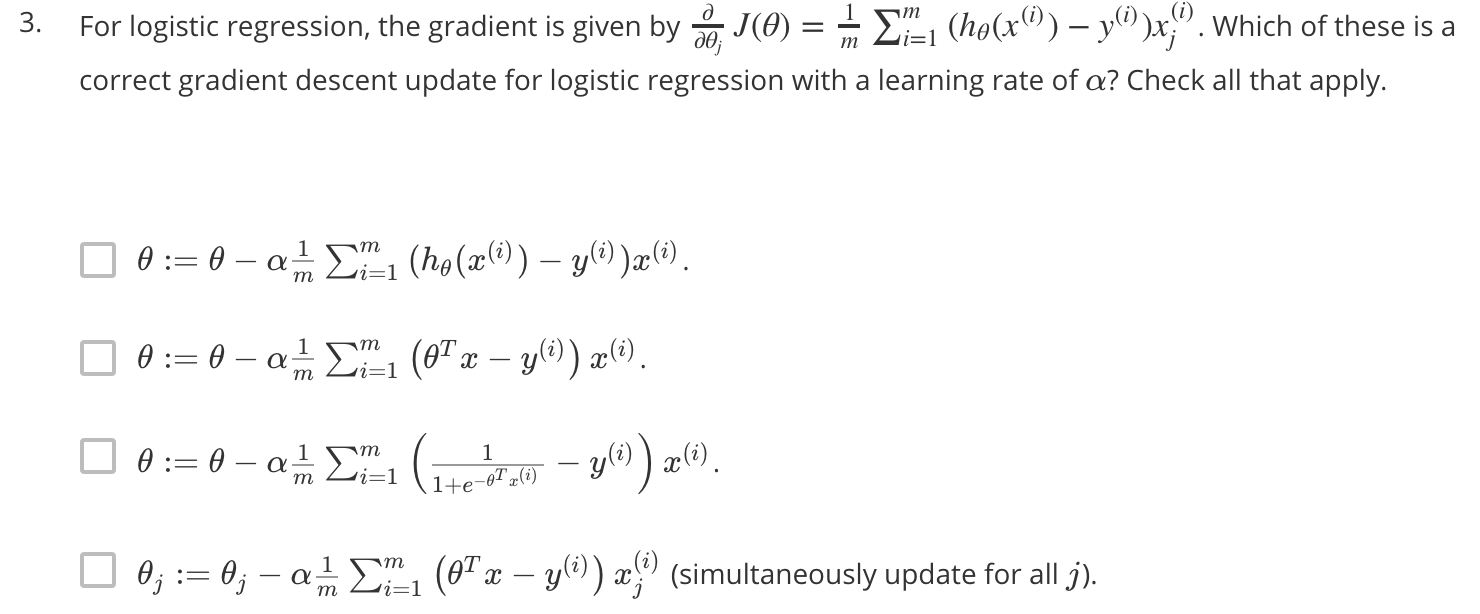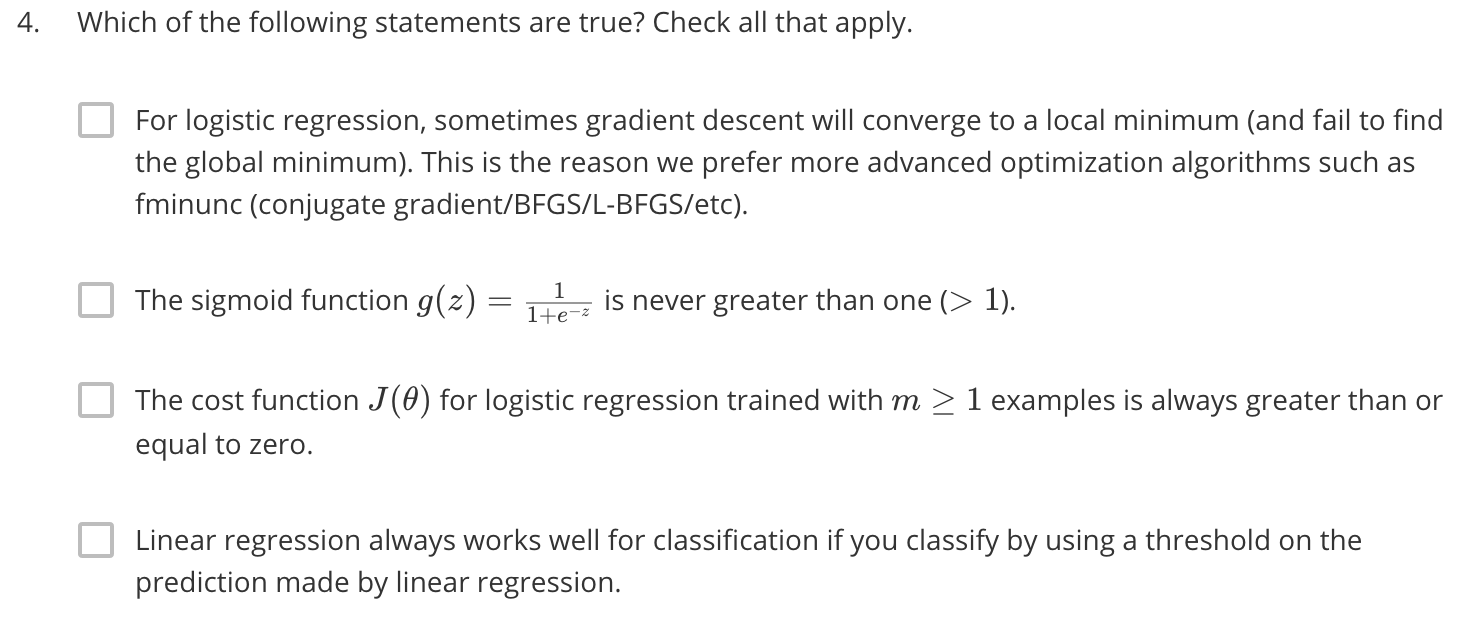Answer:第二项和第三项，对于第一项，这些高级方法的优点在于学习率$\alpha$不用再自己选择，第四项在逻辑回归的开头引出的时候讲了，线性回归的缺陷就在那里。